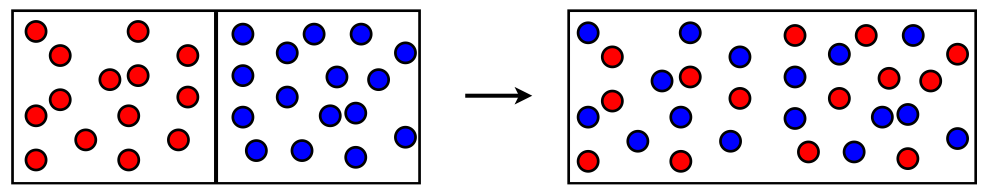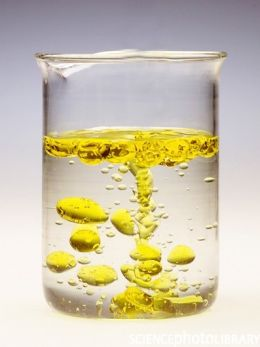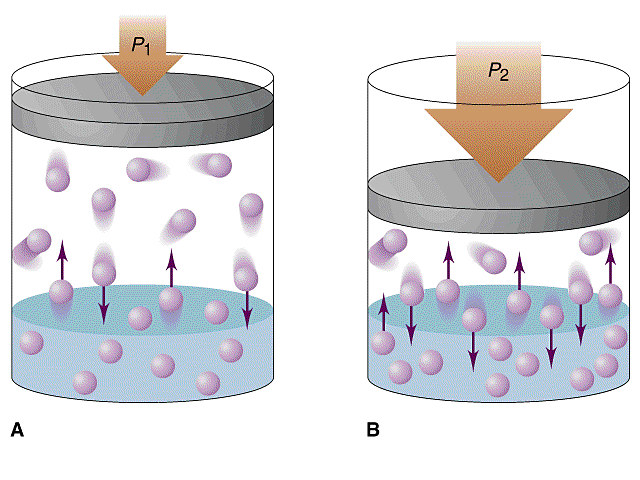### Solutions

Solutions are homogeneous mixtures typically composed of a major component that is a liquid and a minor component that is dissolved in the liquid.   The major component is called the solvent and the minor component(s) is(are) the solute(s).   A key idea in thinking molecularly about a solution is to imagine the intermolecular forces between the components.   Since the solvent is the majority of the mixture, its intermolecular forces are very similar to the pure substance.   This is because each solvent molecule is typically surrounded by other solvent molecules.  Only every once in a while is a solvent molecule next to a solute molecule.   And even then, it is only one.  On the otherhand, the IMF for the solute are extremely different in the solution than in the pure substance.   This is because now the solute is completely surrounded by solvent (while before it was surrounded by itself).   This makes compounds in solutions very different then their pure substances.  As such in a chemical reaction, we denote these as if they are a different "phase".   Much of the chemistry this semester will focus on aqueous solutions. These are solutions in which water is the solvent.   When writing a chemical reaction we denote something that is in an aqueous solution with (aq).  Just as we would denote other substances as (g) or (s) as gases or solids.

Solutions

### mixtures

To understand solutions, it is important to understand a few key microscopic ideas about mixtures.  To keep things simple, first imagine a mixture of ideal gases.  This is an easy mixture to approach since for an ideal gas there are no intermolecular forces (IMFs).

Imagine we have two gases in separate containers with a wall in-between.  We remove the wall and the two gases will spontaneously mix.This process will happen spontaneously at constant temperature and pressure.  This means that there is a decrease in the free energy ($$\Delta G < 0$$).  There are two reasons the free energy can go down.

$\Delta G = \Delta H - T\Delta S$

Either the enthalpy is going down, or the entropy is going up.  Since we have an ideal gas there are no IMFs.  No IMFs = no potential energy.  With no potential energy there can be no change in potential energy.  No change in potential energy means that the enthalpy is constant ($$\Delta H = 0$$).

Therefore the entropy must be going up.  This is always the case for mixing.  $$\rm\Delta S_{mixing}>0$$.  That is, mixing increases the entropy!

What about a system with IMFs?  Then $$\Delta H$$ depends a lot on what the two substances are.  The change in enthalpy depends on the difference between the IMFs of the two compounds that are being mixed.  If you can't remember your IMFs, review them now (opens in a new tab or window) from our CH301 eBook site.

A solution is ideal if $$\Delta H = 0$$ for forming the solution.  This does not mean there are no IMFs (as is the case for gases), but rather that there is no change in IMFs.  For this to be true, the IMFs between the solvent and the solute need to be identical to the solvent and solute with themselves.   Generally solutions are not ideal, but often we will imagine them as ideal to understand what effects are the result of entropy and what effects depend on the enthalpy.

### enthalpy of solution

Let’s look at how the IMFs are changed during the formation of the solution.

There are two key parts to the changes in the forces. The largest changes are for the solute. Before mixing, the solute molecules only interact with other solute molecules. In the solution, the solute molecules only interact with solvent molecules. Thus the changes result from the loss of the solute-solute interaction and the gain of the solute- solvent interactions. (It should be noted that along with this, there is a small loss of solvent-solvent interactions.)

We can write this out as

$\Delta H_{\rm solution} = \Delta H_{\rm lattice\;energy} + \Delta H_{\rm solvation}$

The first term is the change in enthalpy on forming the solution. The other two terms are the change broken down into the two changes discussed in the details above. The loss of solute-solute interaction and the gain in solute-solvent interactions. The first term is noted here as the Lattice Energy. This is what we typically associate with the energy of forming an ionic solid from separated ions. This is the energy required to pull the solute apart. The second term is the enthalpy of solvation. This is the energy released when the solute interacts with the solvent.  As we are often talking about solutions made with water as the solvent, this term is often referred to as the enthalpy of hydration, $$\Delta H_{\rm hydration}$$.

VERY IMPORTANT NOTE: It is critical that you keep track of the sign of these two enthalpy changes. Often the lattice-energy is a negative number. However, here you need to use it as a positive number. It is the energy input to overcome the solute/solute interactions. Thus you need it as a positive number. Energy in. Positive change.

Likewise the enthalpy of solvation (hydration) should be negative. It is energy out. Negative change.

This is a great example of why understanding is so important. People choose different notations. People choose different sign conventions. You have to know what they mean. Breaking up the solute/solute interactions will always cost energy. Creating the solute/solvent interactions will always release energy.

Now the question remains, which is bigger?

In almost all cases, the lattice energy is larger than the solvation energy.  This means that generally the enthalpy of solution is positive.  It requires energy to dissolve something.  Is this always true?  No.  It is generally true.

### What will dissolve?

So how do we figure out what might dissolve and what won’t? Again we can look at the free energy $$\Delta G = \Delta H - T\Delta S$$.

We have established that generally for forming solutions, $$\Delta H_{\rm solution} > 0$$ and $$\Delta S_{\rm solution} > 0$$. Thus the entropy is helping to lower the free energy, but the enthalpy is raising it.

When will this process be spontaneous?  If the change in free energy is going to be negative, we need

$\left| \,T\Delta S_{\rm solution}\,\right| > \left|\,\Delta H_{\rm solution}\,\right|$

That is, the magnitude of the temperature times the entropy change must be larger than the magnitude of the enthalpy change.  There are two ways we can think about this.  The first is to raise the temperature. This will lead to the entropy term dominating the process.

The second is to make sure the enthalpy term is very small.

How can we do this? We need to make sure that the changes in the intermolecular forces in going from the unmixed solute and solvent to the solution are minimal.

From this, we generate the general idea how to understand what things will dissolve (mix).

You may have heard the phrase “Like Dissolves Like.”

This means that similar things dissolve similar things. What do we mean by similar? Similar intermolecular forces. Similar intermolecular forces between solute and solvent means there isn’t much change in energy upon making the solution. This is what we mean by minimizing $$\Delta H_{\rm solution}$$.

Solvents with hydrogen bonding will dissolve solutes with hydrogen bonding. Solvent with hydrogen bonding will also dissolve ionic solutes (as they have strong electrostatic interactions).

Non-polar solvents will dissolve non-polar solutes. Non-polar solvents will not dissolve polar solutes (or ionic solutes) since they do not have similar types of intermolecular forces

For example, oil does not dissolve into water. Oils are long chain hydrocarbons with only dispersion forces. Water intermolecular forces are dominated by hydrogen bonding. In contrast, sugar dissolves in water. Sugar molecules exhibit hydrogen bonding so it has similar interactions as the water.

### Things that don't dissolve

Things that are very different. Oil and water. Oil has dispersion forces. Water has hydrogen bonding.

However, when is similar not similar enough?  We can get a good idea looking at ionic compounds and water.

Water is polar. It isn’t ionic. So already we know they are not the same. However, dipole-ion forces are substantial even though they are likely not as large as ion-ion forces. Especially since hydrogen bonding interactions are so strong. When will this difference be too much? When the ion-ion forces are really large.

If you remember from studying ionic bonding, the forces between the ions depends on the charge and the separation between the ions. These two ideas can be combined into one: charge density. This is how compact the charge is. When the ion is small and has a large charge (Mg2+), it has a very large charge density. When it is large and has a low charge (I-), it has low charge density. The larger the charge density of the two ions, the stronger the forces holding together the solute and the less likely they are to dissolve. So for example, while NaCl is very soluble in water, MgO is not. Neither is soluble in hexane (a non-polar solvent).

There is a second complication with large charge density ions. Remember the entropy term we said was amost always positive for mixing. One of the reasons for the “almost” is high charge density ions. When they dissolve into solution they interact very strongly with the water molecules. This strong interaction that leads to the water essentially clinging to the ion, reduces the entropy of the water. Before it was wandering all over. Now it is stuck to the ion. As such, the entropy of solution for high charge density ions is negative! Now we have a situation were enthalpy is very large (that is bad for making a solution) and the entropy is negative (that is also bad for making a solution). Nothing is in favor of dissolving. Thus these compounds are really really insoluble.

### Mixture of liquids (miscibility)

Miscibility refers to making a solution of liquids.

If two liquids mix in any proportions we call them miscible.

If they don’t they are immiscible.The picture  shows two immiscible liquids. These are liquids with very different IMFs, for example oil (long hydrocarbon chain, non-polar) and water (polar, H-bonding).  Again, “like dissolves like,” “like is miscible with like.”

When you are deciding what molecules are alike, look at the intermolecular forces. For example, CH3OH (methanol) is an alcohol that is miscible with water. Both are polar and both have H-bonds. However, you might think that methanol is more like CH4 (methane) since it is only different due to the missing oxygen atom. However methane is completely different. It is non-polar and has very weak dispersion forces. As such it is a gas not a liquid! It is also only sparingly soluble in water.

### Mixtures of liquids and gases (Henry's Law)

When gases dissolve into liquids we have a slightly different situation from the dissolution of a solid or a liquid into another liquid. The biggest issue is the concentration of the "pure" solute. In the case of the solid or a liquid the pure substance is the pure substance. The "molecules" are all next to each other and the concentration is the same at all conditions. For a gas, the "pure" substance has a concentration that is extremely dependent on the conditions. Thus the most important factor for a gas dissolving into a liquid is the concentration of the gas above the liquid. In chemistry we express concentrations of gases as partial pressure. The solubility of gas depends on the partial pressure of the gas above the solvent.

The solubility of a gas in the liquid is quantified using Henry’s Law.  Henry’s Law states that the mole fraction of gas dissolved in the liquid (like the concentration) is directly proportional to the pressure of the gas over the liquid.

$P_{\rm gas} = K X_{\rm gas}$

The constant K depends on the IMFs between the solute and the solvent and thus it depends on both the chemical structure of the gas and the liquid.   Xgas is the mole fraction which is the (moles of gas)/(total number of moles of the mixture).

Henry's Law is sometimes given with different units (but it is exactly the same otherwise).  You may find it written as

$C_{\rm gas} = k_{\rm H} \; P_{\rm gas}$

Here the Henry's Law constant has units of M/atm.   It might also be written as above, but with molarity instead of mole fraction.   You might also find it given as the 2nd equation but with mole fraction instead of molarity. Or any number of other ways!  Watch the units.  (and note that mole fraction has no units).

The consequences of Henry's Law are fairly straight forward. Double the pressure.  Double the concentration (mole fraction).Gases dissolved in liquids seems a bit of a random topic, but in fact Henry’s Law shows up in every day life.   Have you ever wondered how they get all the CO2 into carbonated beverages?  They apply a big pressure of CO2 above the liquid and the CO2 dissolves.  When the pressure goes away (the pssst when you open the can), the amount of CO2 dissolved decreases and bubbles appear.

Also, if you are a scuba diver, you know you do not come up from great depths too quickly.  This is because when your body is at higher pressure, N2 dissolves into your blood and tissue.  Should you come up to the surface too quickly the pressure drops and, just like the soda can, bubbles form.  N2 bubbles in your body can be very painful. Worse, bubbles in your brain tissue and spinal cord are very bad for your health.

Finally, Henry’s law is important for fish (and marine life in general).   Fish, if you didn’t know, manage to get O2 out of the water to live.  As the earth’s atmosphere has a partial pressure of oxygen of around 0.18 atm there is always some O2 dissolved in water (rivers, lakes, oceans, the glass of water you are drinking,…)  However, as we will discuss later the temperature dependence of solubility depends on the enthalpy. The fact that dissolution of gases is exothermic means that their solubility decreases with increasing temperature. Thus rising ocean temperatures mean lower dissolved O2 levels. This is turn leads to tough times for marine life.

Note: This is a very easy (if a bit boring) experiment to do at home.  Put a pan of water on the stove and heat it up.  What happens?  Way before the solution begins to boil, little bubbles form on the bottom of the pan. Why?  This is the dissolved air coming out of the water.  As you raise the temperature the solubility drops and the gas comes out. (Note: The bubbles tend to form in the same place. This is because the surface tension of water makes the bubble formation slow.  So the bubbles will form at little defects or scratches in the bottom of your pan.) Alternatively (and more amusing) open a very cold can of soda and a very warm can of soda and notice the difference in the solubility of the CO2 in the two situations.

### More details

Other differences for gases dissolving into liquids. For a gas dissolving in a liquid the entropy of solution is negative, $$\Delta S _{\rm solution} < 0$$. The solute is in the gas phase which is the highest entropy state.  So even though we are making a mixture, the entropy will be going down. A solution will form only if $$\Delta G _{\rm solution} < 0$$. However, now the entropy term is causing the free energy to increase. The only way that we can a have a decrease in the free energy is if the solution process is exothermic. This is in fact the gas for gas dissolution. The reason is a key difference for the enthlapy of gases compared to other solutes. For gases we don’t have to overcome any intermolecular forces in pulling the solute molecules away from each other.  They are in the gas phase and already apart.  Thus $$\Delta H _{\rm solution} = \Delta H_{\rm solvation}$$ since the lattice-energy term is zero (because it is a gas with no IMFs).   Since the solvation term is negative, we find that $$\Delta H_{\rm solution} < 0$$.   A solution will form to a small extent thanks to the enthalpy term being exothermic.

Henry's Law